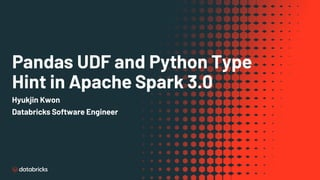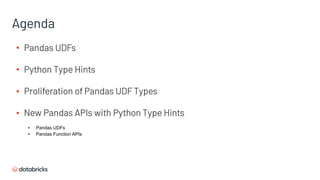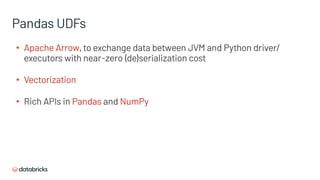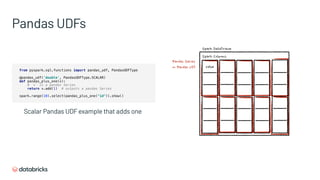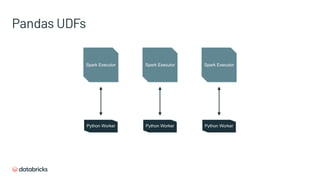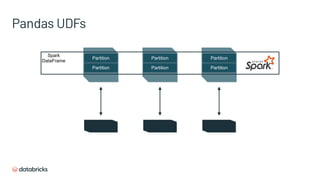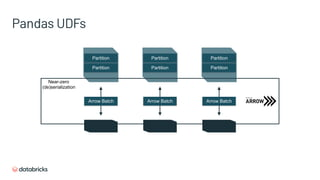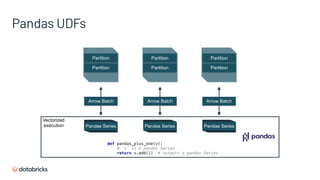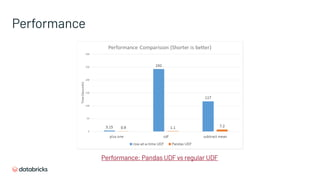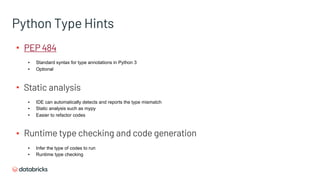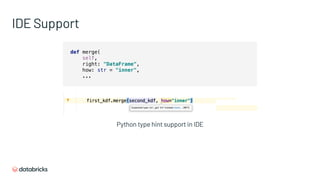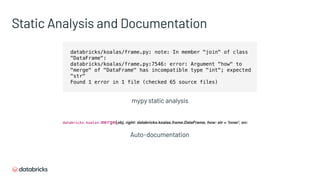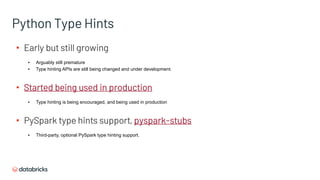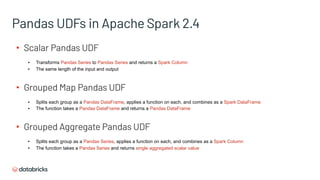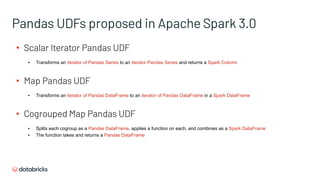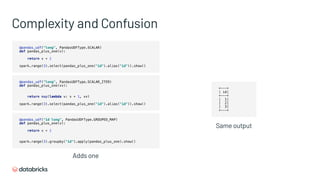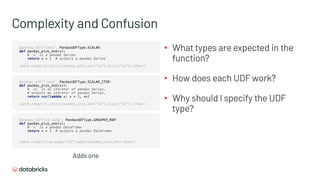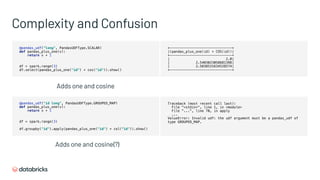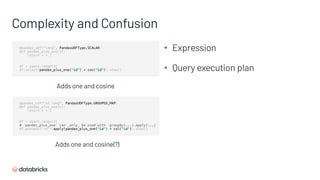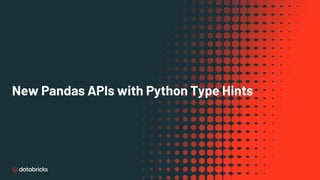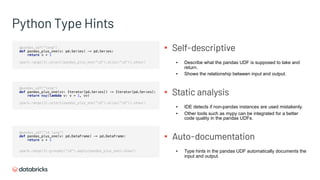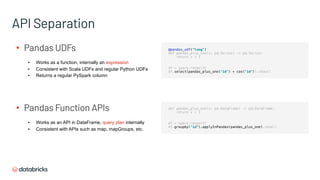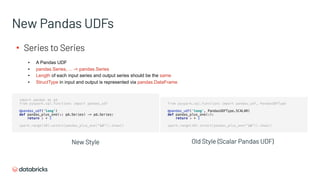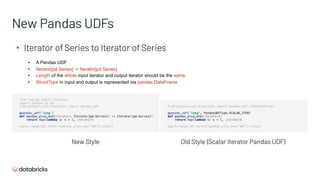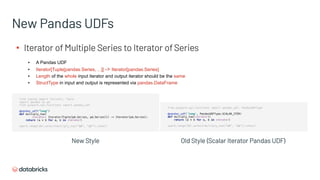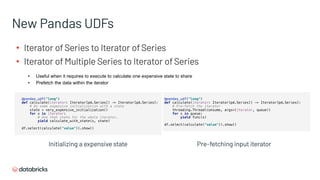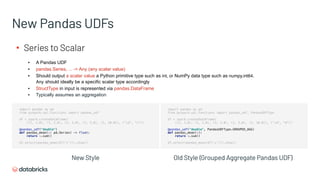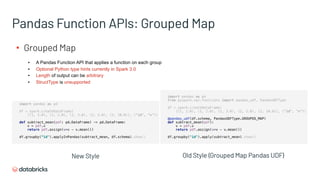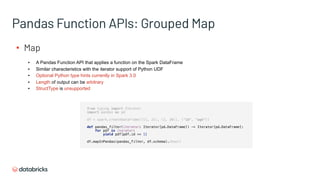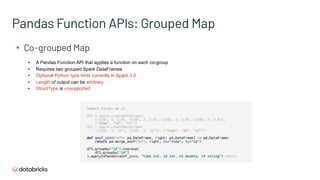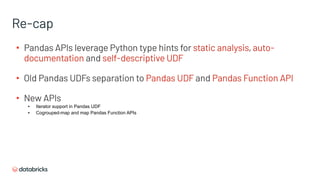1 of 38

### Pandas UDF and Python Type Hint in Apache Spark 3.0

1. Pandas UDF and Python Type Hint in Apache Spark 3.0 Hyukjin Kwon Databricks Software Engineer
2. Hyukjin Kwon ▪ Apache Spark PMC / Committer ▪ Major Koalas contributor ▪ Databricks Software Engineer ▪ @HyukjinKwon in Github
3. Agenda ▪ Pandas UDFs ▪ Python Type Hints ▪ Proliferation of Pandas UDF Types ▪ New Pandas APIs with Python Type Hints ▪ Pandas UDFs ▪ Pandas Function APIs
4. Pandas UDFs
5. Pandas UDFs ▪ Apache Arrow, to exchange data between JVM and Python driver/ executors with near-zero (de)serialization cost ▪ Vectorization ▪ Rich APIs in Pandas and NumPy
6. Pandas UDFs from pyspark.sql.functions import pandas_udf, PandasUDFType @pandas_udf('double', PandasUDFType.SCALAR) def pandas_plus_one(v): # `v` is a pandas Series return v.add(1) # outputs a pandas Series spark.range(10).select(pandas_plus_one("id")).show() Scalar Pandas UDF example that adds one Spark DataFrame Spark Columns Value Pandas Series in Pandas UDF
7. Pandas UDFs Spark Executor Spark Executor Spark Executor Python Worker Python Worker Python Worker
8. Pandas UDFs Partition Partition Partition Partition Partition Partition Spark DataFrame
9. Pandas UDFs Partition Partition Partition Partition Partition Partition Arrow Batch Arrow Batch Arrow Batch Near-zero (de)serialization
10. Pandas UDFs Partition Partition Partition Partition Partition Partition Arrow Batch Pandas Series Arrow Batch Arrow Batch Pandas Series Pandas Series def pandas_plus_one(v): # `v` is a pandas Series return v.add(1) # outputs a pandas Series Vectorized execution
11. Performance Performance: Pandas UDF vs regular UDF
12. Python Type Hints
13. Python Type Hints def greeting(name): return 'Hello ' + name Typical Python codes def greeting(name: str) -> str: return 'Hello ' + name Python codes with type hints
14. Python Type Hints ▪ PEP 484 ▪ Standard syntax for type annotations in Python 3 ▪ Optional ▪ Static analysis ▪ IDE can automatically detects and reports the type mismatch ▪ Static analysis such as mypy ▪ Easier to refactor codes ▪ Runtime type checking and code generation ▪ Infer the type of codes to run ▪ Runtime type checking
15. IDE Support def merge( self, right: "DataFrame", how: str = "inner", ... Python type hint support in IDE
16. Static Analysis and Documentation databricks/koalas/frame.py: note: In member "join" of class "DataFrame": databricks/koalas/frame.py:7546: error: Argument "how" to "merge" of "DataFrame" has incompatible type "int"; expected "str" Found 1 error in 1 file (checked 65 source files) mypy static analysis Auto-documentation
17. Python Type Hints ▪ Early but still growing ▪ Arguably still premature ▪ Type hinting APIs are still being changed and under development. ▪ Started being used in production ▪ Type hinting is being encouraged, and being used in production ▪ PySpark type hints support, pyspark-stubs ▪ Third-party, optional PySpark type hinting support.
18. Proliferation of Pandas UDF Types
19. Pandas UDFs in Apache Spark 2.4 ▪ Scalar Pandas UDF ▪ Transforms Pandas Series to Pandas Series and returns a Spark Column ▪ The same length of the input and output ▪ Grouped Map Pandas UDF ▪ Splits each group as a Pandas DataFrame, applies a function on each, and combines as a Spark DataFrame ▪ The function takes a Pandas DataFrame and returns a Pandas DataFrame ▪ Grouped Aggregate Pandas UDF ▪ Splits each group as a Pandas Series, applies a function on each, and combines as a Spark Column ▪ The function takes a Pandas Series and returns single aggregated scalar value
20. Pandas UDFs proposed in Apache Spark 3.0 ▪ Scalar Iterator Pandas UDF ▪ Transforms an iterator of Pandas Series to an iterator Pandas Series and returns a Spark Column ▪ Map Pandas UDF ▪ Transforms an iterator of Pandas DataFrame to an iterator of Pandas DataFrame in a Spark DataFrame ▪ Cogrouped Map Pandas UDF ▪ Splits each cogroup as a Pandas DataFrame, applies a function on each, and combines as a Spark DataFrame ▪ The function takes and returns a Pandas DataFrame
21. Complexity and Confusion @pandas_udf("long", PandasUDFType.SCALAR) def pandas_plus_one(v): return v + 1 spark.range(3).select(pandas_plus_one("id").alias("id")).show() @pandas_udf("long", PandasUDFType.SCALAR_ITER) def pandas_plus_one(vv): return map(lambda v: v + 1, vv) spark.range(3).select(pandas_plus_one("id").alias("id")).show() @pandas_udf("id long", PandasUDFType.GROUPED_MAP) def pandas_plus_one(v): return v + 1 spark.range(3).groupby("id").apply(pandas_plus_one).show() +---+ | id| +---+ | 1| | 2| | 3| +---+ Same output Adds one
22. Complexity and Confusion @pandas_udf("long", PandasUDFType.SCALAR) def pandas_plus_one(v): # `v` is a pandas Series return v + 1 # outputs a pandas Series spark.range(3).select(pandas_plus_one("id").alias("id")).show() @pandas_udf("long", PandasUDFType.SCALAR_ITER) def pandas_plus_one(vv): # `vv` is an iterator of pandas Series. # outputs an iterator of pandas Series. return map(lambda v: v + 1, vv) spark.range(3).select(pandas_plus_one("id").alias("id")).show() @pandas_udf("id long", PandasUDFType.GROUPED_MAP) def pandas_plus_one(v): # `v` is a pandas DataFrame return v + 1 # outputs a pandas DataFrame spark.range(3).groupby("id").apply(pandas_plus_one).show() ▪ What types are expected in the function? ▪ How does each UDF work? ▪ Why should I specify the UDF type? Adds one
23. Complexity and Confusion @pandas_udf("long", PandasUDFType.SCALAR) def pandas_plus_one(v): return v + 1 df = spark.range(3) df.select(pandas_plus_one("id") + cos("id")).show() @pandas_udf("id long", PandasUDFType.GROUPED_MAP) def pandas_plus_one(v): return v + 1 df = spark.range(3) df.groupby("id").apply(pandas_plus_one("id") + col(“id")).show() Adds one and cosine Adds one and cosine(?) Traceback (most recent call last): File "<stdin>", line 1, in <module> File "...", line 70, in apply ... ValueError: Invalid udf: the udf argument must be a pandas_udf of type GROUPED_MAP. +-------------------------------+ |(pandas_plus_one(id) + COS(id))| +-------------------------------+ | 2.0| | 2.5403023058681398| | 2.5838531634528574| +-------------------------------+
24. Complexity and Confusion @pandas_udf("long", PandasUDFType.SCALAR) def pandas_plus_one(v): return v + 1 df = spark.range(3) df.select(pandas_plus_one("id") + cos("id")).show() @pandas_udf("id long", PandasUDFType.GROUPED_MAP) def pandas_plus_one(v): return v + 1 df = spark.range(3) # `pandas_plus_one` can _only_ be used with `groupby(...).apply(...)` df.groupby("id").apply(pandas_plus_one("id") + col("id")).show() Adds one and cosine Adds one and cosine(?) ▪ Expression ▪ Query execution plan
25. New Pandas APIs with Python Type Hints
26. Python Type Hints @pandas_udf("long") def pandas_plus_one(v: pd.Series) -> pd.Series: return v + 1 spark.range(3).select(pandas_plus_one("id").alias("id")).show() @pandas_udf("long") def pandas_plus_one(vv: Iterator[pd.Series]) -> Iterator[pd.Series]: return map(lambda v: v + 1, vv) spark.range(3).select(pandas_plus_one("id").alias("id")).show() @pandas_udf("id long") def pandas_plus_one(v: pd.DataFrame) -> pd.DataFrame: return v + 1 spark.range(3).groupby("id").apply(pandas_plus_one).show() ▪ Self-descriptive ▪ Describe what the pandas UDF is supposed to take and return. ▪ Shows the relationship between input and output. ▪ Static analysis ▪ IDE detects if non-pandas instances are used mistakenly. ▪ Other tools such as mypy can be integrated for a better code quality in the pandas UDFs. ▪ Auto-documentation ▪ Type hints in the pandas UDF automatically documents the input and output.
27. ▪ Pandas UDFs ▪ Works as a function, internally an expression ▪ Consistent with Scala UDFs and regular Python UDFs ▪ Returns a regular PySpark column ▪ Pandas Function APIs ▪ Works as an API in DataFrame, query plan internally ▪ Consistent with APIs such as map, mapGroups, etc. API Separation @pandas_udf("long") def pandas_plus_one(v: pd.Series) -> pd.Series: return v + 1 df = spark.range(3) df.select(pandas_plus_one("id") + cos("id")).show() def pandas_plus_one(v: pd.DataFrame) -> pd.DataFrame: return v + 1 df = spark.range(3) df.groupby("id").applyInPandas(pandas_plus_one).show()
28. ▪ Series to Series ▪ A Pandas UDF ▪ pandas.Series, ... -> pandas.Series ▪ Length of each input series and output series should be the same ▪ StructType in input and output is represented via pandas.DataFrame New Pandas UDFs import pandas as pd from pyspark.sql.functions import pandas_udf @pandas_udf('long') def pandas_plus_one(s: pd.Series) -> pd.Series: return s + 1 spark.range(10).select(pandas_plus_one("id")).show() New Style from pyspark.sql.functions import pandas_udf, PandasUDFType @pandas_udf('long', PandasUDFType.SCALAR) def pandas_plus_one(v): return v + 1 spark.range(10).select(pandas_plus_one("id")).show() Old Style (Scalar Pandas UDF)
29. New Pandas UDFs ▪ Iterator of Series to Iterator of Series ▪ A Pandas UDF ▪ Iterator[pd.Series] -> Iterator[pd.Series] ▪ Length of the whole input iterator and output iterator should be the same ▪ StructType in input and output is represented via pandas.DataFrame from typing import Iterator import pandas as pd from pyspark.sql.functions import pandas_udf @pandas_udf('long') def pandas_plus_one(iterator: Iterator[pd.Series]) -> Iterator[pd.Series]: return map(lambda s: s + 1, iterator) spark.range(10).select(pandas_plus_one("id")).show() New Style from pyspark.sql.functions import pandas_udf, PandasUDFType @pandas_udf('long', PandasUDFType.SCALAR_ITER) def pandas_plus_one(iterator): return map(lambda s: s + 1, iterator) spark.range(10).select(pandas_plus_one("id")).show() Old Style (Scalar Iterator Pandas UDF)
30. New Pandas UDFs ▪ Iterator of Multiple Series to Iterator of Series ▪ A Pandas UDF ▪ Iterator[Tuple[pandas.Series, ...]] -> Iterator[pandas.Series] ▪ Length of the whole input iterator and output iterator should be the same ▪ StructType in input and output is represented via pandas.DataFrame from typing import Iterator, Tuple import pandas as pd from pyspark.sql.functions import pandas_udf @pandas_udf("long") def multiply_two( iterator: Iterator[Tuple[pd.Series, pd.Series]]) -> Iterator[pd.Series]: return (a * b for a, b in iterator) spark.range(10).select(multiply_two("id", "id")).show() New Style from pyspark.sql.functions import pandas_udf, PandasUDFType @pandas_udf('long', PandasUDFType.SCALAR_ITER) def multiply_two(iterator): return (a * b for a, b in iterator) spark.range(10).select(multiply_two("id", "id")).show() Old Style (Scalar Iterator Pandas UDF)
31. New Pandas UDFs ▪ Iterator of Series to Iterator of Series ▪ Iterator of Multiple Series to Iterator of Series ▪ Useful when it requires to execute to calculate one expensive state to share ▪ Prefetch the data within the iterator @pandas_udf("long") def calculate(iterator: Iterator[pd.Series]) -> Iterator[pd.Series]: # Do some expensive initialization with a state state = very_expensive_initialization() for x in iterator: # Use that state for the whole iterator. yield calculate_with_state(x, state) df.select(calculate("value")).show() Initializing a expensive state @pandas_udf("long") def calculate(iterator: Iterator[pd.Series]) -> Iterator[pd.Series]: # Pre-fetch the iterator threading.Thread(consume, args=(iterator, queue)) for s in queue: yield func(s) df.select(calculate("value")).show() Pre-fetching input iterator
32. New Pandas UDFs ▪ Series to Scalar ▪ A Pandas UDF ▪ pandas.Series, ... -> Any (any scalar value) ▪ Should output a scalar value a Python primitive type such as int, or NumPy data type such as numpy.int64.  Any should ideally be a specific scalar type accordingly ▪ StructType in input is represented via pandas.DataFrame ▪ Typically assumes an aggregation import pandas as pd from pyspark.sql.functions import pandas_udf df = spark.createDataFrame( [(1, 1.0), (1, 2.0), (2, 3.0), (2, 5.0), (2, 10.0)], ("id", "v")) @pandas_udf("double") def pandas_mean(v: pd.Series) -> float: return v.sum() df.select(pandas_mean(df['v'])).show() New Style import pandas as pd from pyspark.sql.functions import pandas_udf, PandasUDFType df = spark.createDataFrame( [(1, 1.0), (1, 2.0), (2, 3.0), (2, 5.0), (2, 10.0)], ("id", "v")) @pandas_udf("double", PandasUDFType.GROUPED_AGG) def pandas_mean(v): return v.sum() df.select(pandas_mean(df['v'])).show() Old Style (Grouped Aggregate Pandas UDF)
33. Pandas Function APIs: Grouped Map ▪ Grouped Map ▪ A Pandas Function API that applies a function on each group ▪ Optional Python type hints currently in Spark 3.0 ▪ Length of output can be arbitrary ▪ StructType is unsupported import pandas as pd df = spark.createDataFrame( [(1, 1.0), (1, 2.0), (2, 3.0), (2, 5.0), (2, 10.0)], ("id", "v")) def subtract_mean(pdf: pd.DataFrame) -> pd.DataFrame: v = pdf.v return pdf.assign(v=v - v.mean()) df.groupby(“id").applyInPandas(subtract_mean, df.schema).show() New Style import pandas as pd from pyspark.sql.functions import pandas_udf, PandasUDFType df = spark.createDataFrame( [(1, 1.0), (1, 2.0), (2, 3.0), (2, 5.0), (2, 10.0)], ("id", "v")) @pandas_udf(df.schema, PandasUDFType.GROUPED_MAP) def subtract_mean(pdf): v = pdf.v return pdf.assign(v=v - v.mean()) df.groupby("id").apply(subtract_mean).show() Old Style (Grouped Map Pandas UDF)
34. Pandas Function APIs: Grouped Map ▪ Map ▪ A Pandas Function API that applies a function on the Spark DataFrame ▪ Similar characteristics with the iterator support of Python UDF ▪ Optional Python type hints currently in Spark 3.0 ▪ Length of output can be arbitrary ▪ StructType is unsupported from typing import Iterator import pandas as pd df = spark.createDataFrame([(1, 21), (2, 30)], ("id", "age")) def pandas_filter(iterator: Iterator[pd.DataFrame]) -> Iterator[pd.DataFrame]: for pdf in iterator: yield pdf[pdf.id == 1] df.mapInPandas(pandas_filter, df.schema).show()
35. Pandas Function APIs: Grouped Map ▪ Co-grouped Map ▪ A Pandas Function API that applies a function on each co-group ▪ Requires two grouped Spark DataFrames ▪ Optional Python type hints currently in Spark 3.0 ▪ Length of output can be arbitrary ▪ StructType is unsupported import pandas as pd df1 = spark.createDataFrame( [(1201, 1, 1.0), (1201, 2, 2.0), (1202, 1, 3.0), (1202, 2, 4.0)], ("time", "id", "v1")) df2 = spark.createDataFrame( [(1201, 1, "x"), (1201, 2, "y")], ("time", "id", "v2")) def asof_join(left: pd.DataFrame, right: pd.DataFrame) -> pd.DataFrame: return pd.merge_asof(left, right, on="time", by="id") df1.groupby("id").cogroup( df2.groupby("id") ).applyInPandas(asof_join, "time int, id int, v1 double, v2 string").show()
36. Re-cap ▪ Pandas APIs leverage Python type hints for static analysis, auto- documentation and self-descriptive UDF ▪ Old Pandas UDFs separation to Pandas UDF and Pandas Function API ▪ New APIs ▪ Iterator support in Pandas UDF ▪ Cogrouped-map and map Pandas Function APIs
37. Questions?